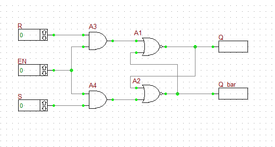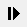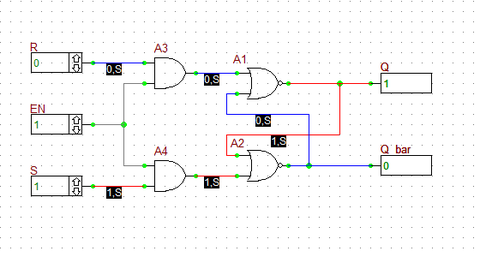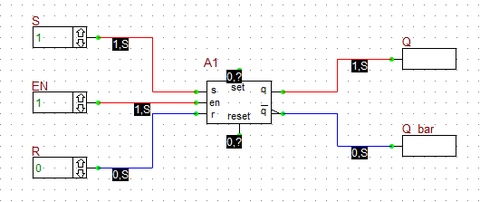# Basic Tutorial Lesson 9: Analyzing a Sequential Logic Circuit - The SR Latch

 Tutorial Project: Analyzing a Sequential Logic Circuit - The SR LatchObjective: In this project, you will use logic gates to build simple sequential logic circuits like SR latches. Concepts/Features: Logic Gates NOR Gate Truth Table Illegal State Minimum Version Required: All versions

## What You Will Learn

In this tutorial you will build SR flip-flop circuits out of logic gates. You will examine their truth table and use them to verify the operation of RF.Spice's own generic SR latch device.

## Building and Testing a Basic SR Latch

The following is a list of parts needed for this part of the tutorial lesson:

Part Name Part Type Part Value
R Digital Input 1-bit
S Digital Input 1-bit
EN Digital Input 1-bit
Q Digital Output N/A
Q_bar Digital Output N/A
A1 - A2 Generic NOR Gate Defaults
A3 - A4 Generic AND Gate Defaults

First, build the basic SR latch using two cross-coupled NOR gates as shown in the above figure.The SR Latch circuit using logic gates.

Before starting the simulation, manually set the inputs as S = 1 and R = 0. This is the "SET" state of the latch. Run a live digital simulation by manual stepping. Click the "Step"button of the Main Toolbar or simply use the keyboard shortcut Ctrl+H. Note that the Q output changes to 1, while its complement Q_bar changes to 0. Change S to 0. Now, you have S = R = 0. This is the "HOLD" state. Step the simulation, and you will note that the output do not change at this state. Now change R to 1, while S is still at 0. This is the "RESET" state of the latch. Step the simulation and you will see that Q changes to 0 and Q_bar turns 1.The outputs of the basic SR Latch circuit with S = 1 and R = 0.

The truth table of the basic NOR SR latch is given below:

S R Q Notes
0 0 Qprev Hold State
0 1 0 Reset
1 0 1 Set
1 1 X Illegal

## Building and Testing a Gated SR Latch

Next, you will add an "Enable" input to your SR latch using two AND gates as shown in the opposite figure. The EN input is fed into both AND gates. Therefore, as long as EN =0, the output of both AND gates is 0. This leads to S = R = 0, which represents the Hold state of the basic NOR SR latch.The Gated SR Latch circuit using logic gates.

Before you start the simulation, set EN = 0. Then, set S = 1 and R = 0. Start a live digital simulation by manual stepping. Unlike the previous case, the output Q and Q_bar do not change this time. Next, set EN = 1. step the simulation and you will see that outputs change to Q = 1 and Q_bar = 0 (Set state). While EN = 1, change to S = 0 and R = 1. Step through and the gated latch enters its Reset sate with Q = 0 and Q_bar = 1.The outputs of the Gated SR Latch circuit with EN = 1, S =1 and R = 0.

The truth table of the gated NOR SR latch is given below:

EN S R Q Notes
0 X X Qprev Hold State
1 0 0 Qprev Hold State
1 0 1 0 Reset
1 1 0 1 Set
1 1 1 X Illegal

## Verifying RF.Spice's SR Latch Device

in the last part of this tutorial lesson, you will remove the two NOR gates and the two AND gates from you digital circuit and replace the four gates with a generic SR latch device. You can access this part from Menu > Parts > Combinational/Sequential Logic. Connect the input S and R pins and the output Q and Q_bar pins of the device as shown in the figure below. Note that unlike the previous circuit, here the top and bottom inputs are called S and R, respectively. Repeat the same procedure in the last part and change the values of the three inputs S, R, and EN in the same manner. You should be able to reproduce a truth table identical to the previous case.The outputs of the SR Latch device with EN = 1, S =1 and R = 0.